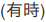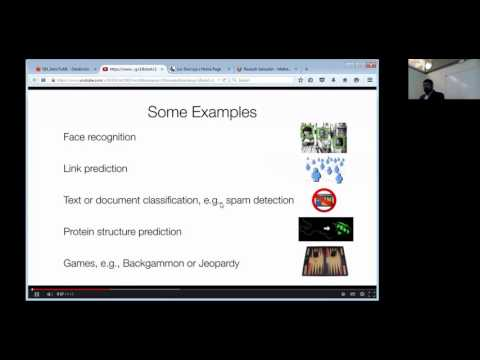// Databricks notebook source exported at Sun, 19 Jun 2016 11:03:13 UTC

# Scalable Data Science

### prepared by Raazesh Sainudiin and Sivanand Sivaram

The html source url of this databricks notebook and its recorded Uji:SOURCE: This is the scala version of the python notebook from the databricks Community Edition that has been added to this databricks shard at Workspace -> scalable-data-science -> xtraResources -> dbCE -> MLlib -> unsupervised -> clustering -> k-means -> 1MSongsPy_ETLExploreModel as extra resources for this project-focussed course Scalable Data Science.

#Data Science with Apache Spark and Scala in Databricks

When you first hear a song, do you ever categorize it as slow or fast in your head? Is it even a valid categorization? If so, can one do it automatically? I have always wondered about that. That is why I got excited when I learned about the Million Songs Dataset.

In this tutorial we will walk through a practical example of a data science project with Apache Spark in Python. We are going to parse, explore and model a sample from the million songs dataset stored on distributed storage. This tutorial is organized into three sections:

1. ETL: Parses raw texts and creates a cached table
2. Explore: Explores different aspects of the songs table using graphs
3. Model: Uses SparkML to cluster songs based on some of their attributesThe goal of this tutorial is to prepare you for real world data science projects. Make sure you go through the tutorial in the above order and use the exercises to make yourself familiar further with the API. Also make sure you run these notebooks on a 1.6.x cluster.

### 1. ETL

The first step of most data science projects is extracting, transforming and loading data into well formated tables. Our example starts with ETL as well. By following the ETL noteboook you can expect to learn about following Spark concepts:

• RDD: Resilient Distributed Dataset
• Schema in Spark
• Spark DataFrame
• Temp tables
• Caching tables

### 2. Explore

Exploratory analysis is a key step in any real data project. Data scientists use variety of tools to explore and visualize their data. In the second notebook of this tutorial we introduce several tools in Python and Databricks notebooks that can help you visually explore your large data. By reading this notebook and finishing its exercises you will become familiar with:

• How to view the schema of a table
• How to display ggplot and matplotlib figures in Notebooks
• How to summarize and visualize different aspects of large datasets
• How to sample and visualize large data

### 3. Model

The end goal of many data scientists is producing useful models. These models are often used for prediction of new and upcoming events in production. In our third notebook we construct a simple K-means clustering model. In this notebook you will learn about:

• Feature transformation
• Fitting a model using SparkML API
• Applying a model to data
• Visualizing model results
• Model tuning

Updated: